# Fraction Calculator – Ultimate Fraction Solver

Use the fraction calculator below to add, subtract, multiply, divide, compare, or simplify fractions.

Fraction One:
Fraction Two:
Enter fractions, numbers, or percents
?
Fraction:

## Result as a Fraction:

1 / 2+3 / 4=11 / 4

## Result as a Decimal:

1 / 2+3 / 4=1.25

### Steps to Solve Expression with Fractions

Find the least common denominator so that all of the denominators match.
The least common denominator of 2 and 4 is 4
Find the multiple for each fraction that can be multiplied by the denominator to get the least common denominator.
Find the multiple by dividing the least common denominator by each fraction's denominator.
Fraction 1 Multiple: 4 ÷ 2 = 2
Fraction 2 Multiple: 4 ÷ 4 = 1
Multiply each fraction's numerator and denominator by the multiple.
1 × 2 / 2 × 2+3 × 1 / 4 × 1=2 / 4+3 / 4
Now that the denominators match, add the numerators
2 + 3 / 4=5 / 4
Reduce the fraction by finding the greatest common factor (GCF) of the numerator and denominator.
GCF of 5 and 4 = 1
Divide the numerator and denominator by the greatest common factor (1).
5 ÷ 1 / 4 ÷ 1=5 / 4
Convert to a mixed fraction by finding the whole number and remainder.
5÷4=1R1
5 / 4=11 / 4
Find the decimal by dividing the numerator by the denominator.
5 / 4=5÷4=1.25
Learn how we calculated this below

## How to Calculate Fractions

A fraction is a numerical value that is not a whole number. It generally represents a portion of a whole, for instance, one slice of a pie.

The calculator above makes it easy to add, subtract, multiply, or divide fractions, and even shows all of the work.

But how do you calculate fractions without a calculator? See the guides below to learn how to add and subtract, multiply, or divide them.

## How to Add & Subtract Fractions

Adding and subtracting fractions is a little different from adding whole numbers. You can use the following formula to add two fractions:

a / b + c / d = ad + bc / bd

Thus, to add two fractions, divide the sum of the product of the numerator of the first fraction a and denominator of the second fraction d and the product of the denominator of the first fraction b and numerator of the second fraction c by the product of the denominators of both fractions b and d.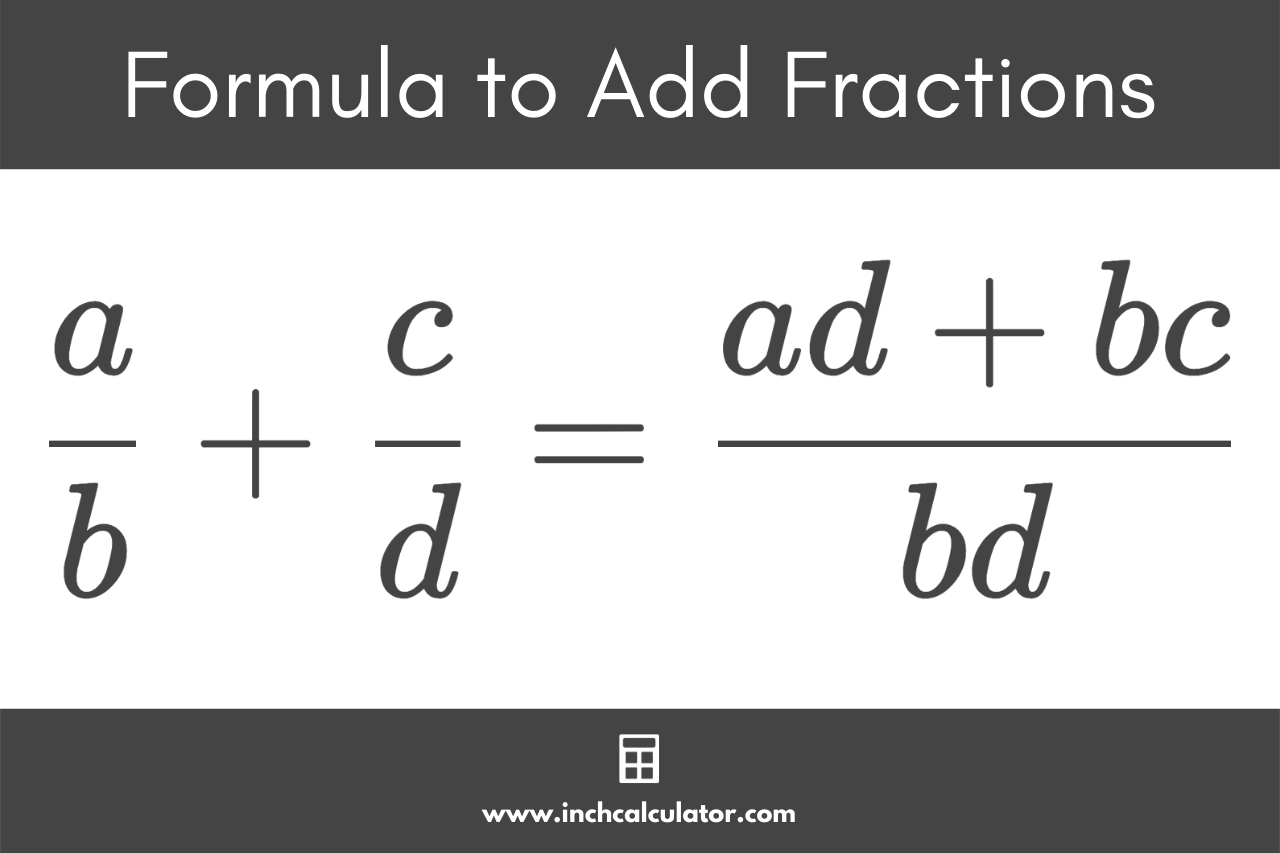This formula is a simple method to make the denominators of both fractions match before adding the numerators. After using the formula, you’ll need to simplify the result.

There are three steps you can use to put this formula to practice to add or subtract fractions.

### Step One: Convert to Fractions with a Common Denominator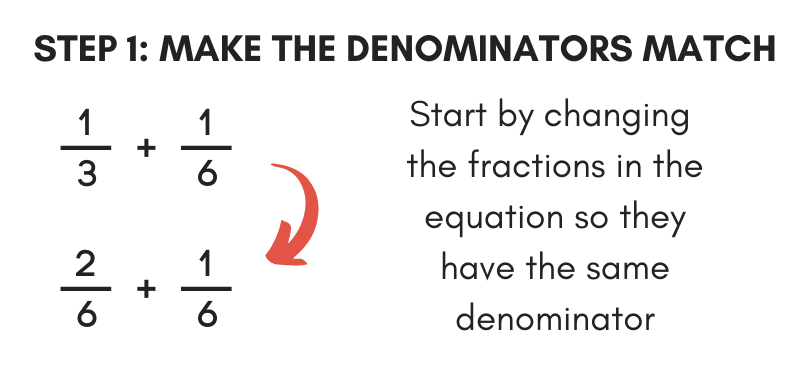The first step is to check the denominators of each fraction. If they have different denominators, then you need to convert them to equivalent fractions with the same denominator.

To do this, start by finding the lowest common denominator for the denominators of both fractions. The lowest common denominator is the smallest number that both denominators can be divided into evenly.

Next, find the multiple for each denominator that, when multiplied by, equals the common denominator. Find the multiple by dividing the common denominator by each denominator.

Then, multiply both the numerator and denominator by the multiple to find the equivalent fractions with matching denominators.

For example, let’s convert the fractions 1 / 3 and 1 / 4 to fractions with the same denominator.

1 / 3 = 1 × 4 / 3 × 4 = 4 / 12
1 / 4 = 1 × 3 / 4 × 3 = 3 / 12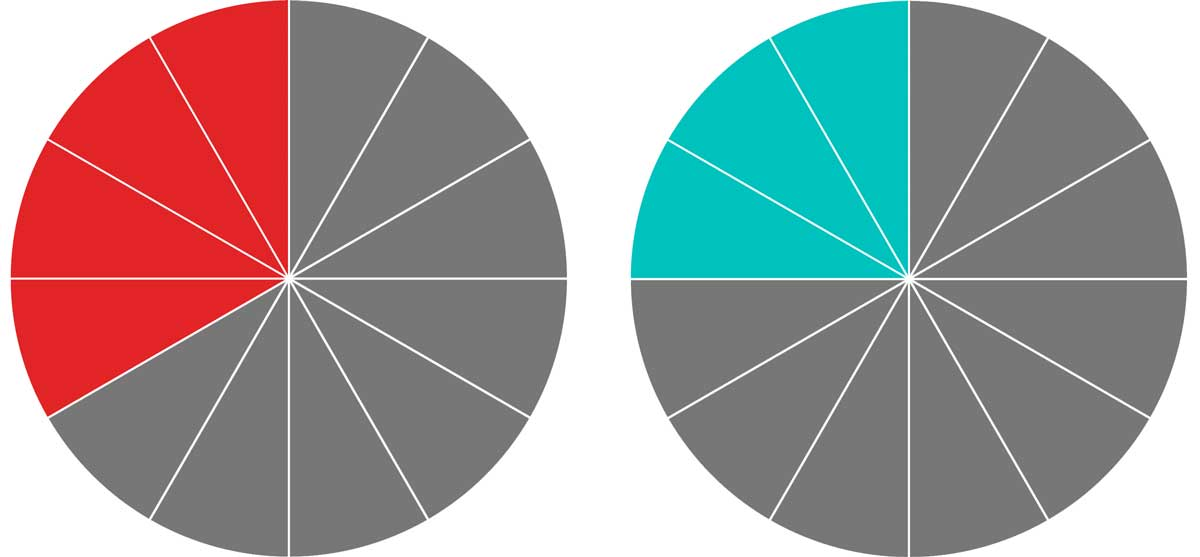### Step Two: Add or Subtract the Numerators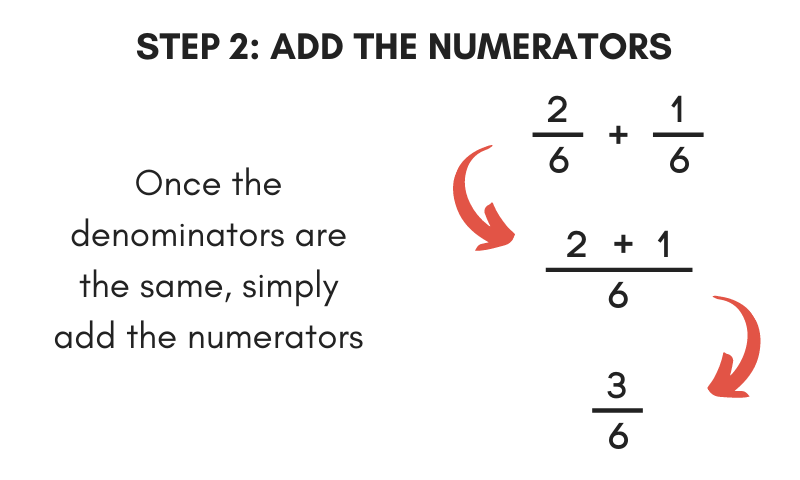Once the denominators are all the same, adding and subtracting fractions is as simple as adding or subtracting the numerators.

To add the fractions, add the numerators together and put them over the common denominator.

To subtract, find the difference between the numerators and put the difference over the common denominator.

For example, continuing the previous example, let’s add 4 / 12 & 3 / 12.

4 / 12 + 3 / 12 = 4 + 3 / 12 = 7 / 12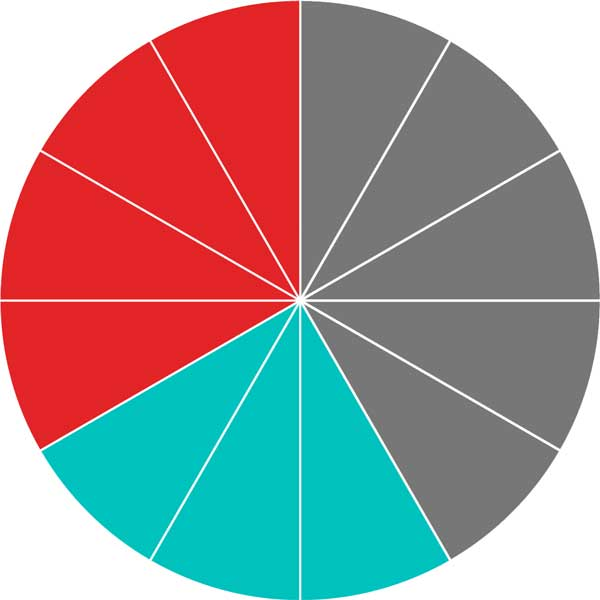### Step Three: Simplify the Fraction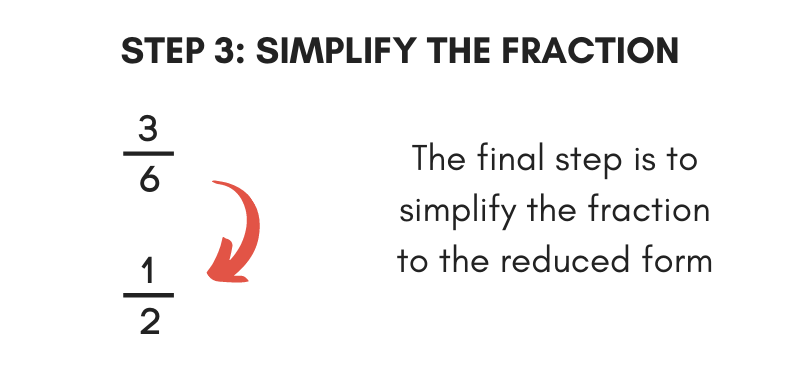The final step to adding or subtracting fractions is to simplify the result fraction. Start by finding the greatest common factor of both the numerator and the denominator. Learn more about finding the greatest common factor for more details.

Then, divide both the numerator and denominator by the greatest common factor to reduce. Or, just use our fraction simplifier to simplify and see all the work needed to do so.

You can also use our adding or subtracting calculators to easily add and subtract fractions.

## How to Multiply Fractions

You can multiply fractions using the following formula:

a / b × c / d = ac / bd

To multiply two fractions, divide the product of the numerators of both fractions a and c by the product of the denominators of both fractions b and d.

Essentially, you just multiply the top numbers of each fraction and multiply the bottom numbers of each fraction.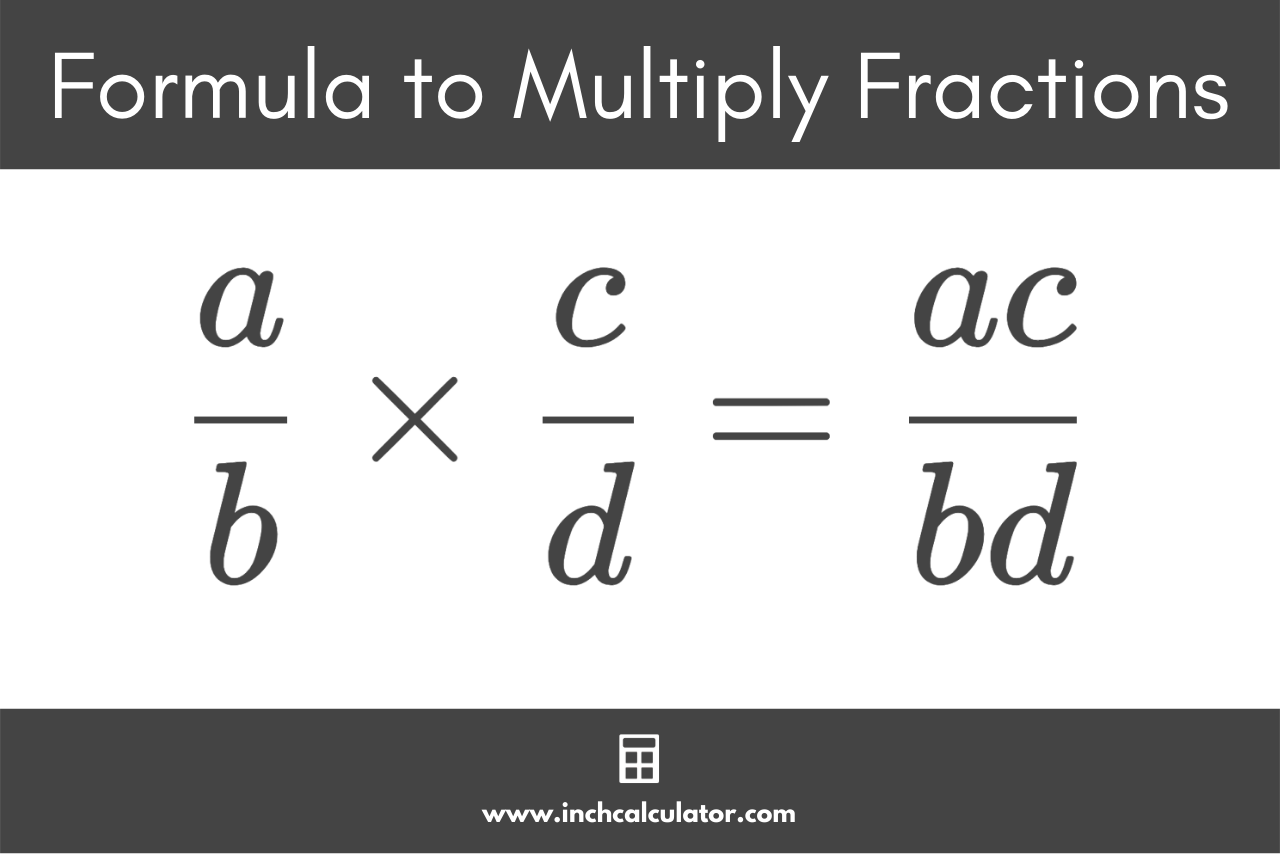Use the following process to multiply two fractions using this formula.

### Step One: Multiply the Numerators and Denominators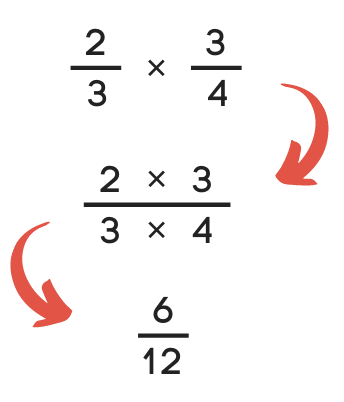The first step is to multiply the numerators together and multiply the denominators together. The result might be an improper fraction, but we’ll reduce it in the next step.

For example, let’s multiply 2 / 3 × 3 / 4.

2 / 3 × 3 / 4 = (2 × 3) / (3 × 4)
2 / 3 × 3 / 4 = 6 / 12

### Step Two: Simplify the Fraction

Like adding and subtracting, the final step of multiplying fractions is to simplify. To simplify, find the numerator and denominator’s greatest common factor, then divide both of them by the common factor.

To simplify 6 / 12, find the greatest common factor.

The greatest common factor of 6 and 12 is 6.

Next, divide both the numerator and denominator by the greatest common factor.

6 / 12 = (6 ÷ 6) / (12 ÷ 6)
6 / 12 = 1 / 2

## How to Divide Fractions

You can divide fractions using the following formula:

a / b ÷ c / d = ad / bc

To divide fractions, divide the product of the first fraction numerator a and second fraction denominator d by the product of the first fraction denominator b and second fraction numerator c.

This is known as cross multiplying because you cross multiply the numerators with the opposite denominators.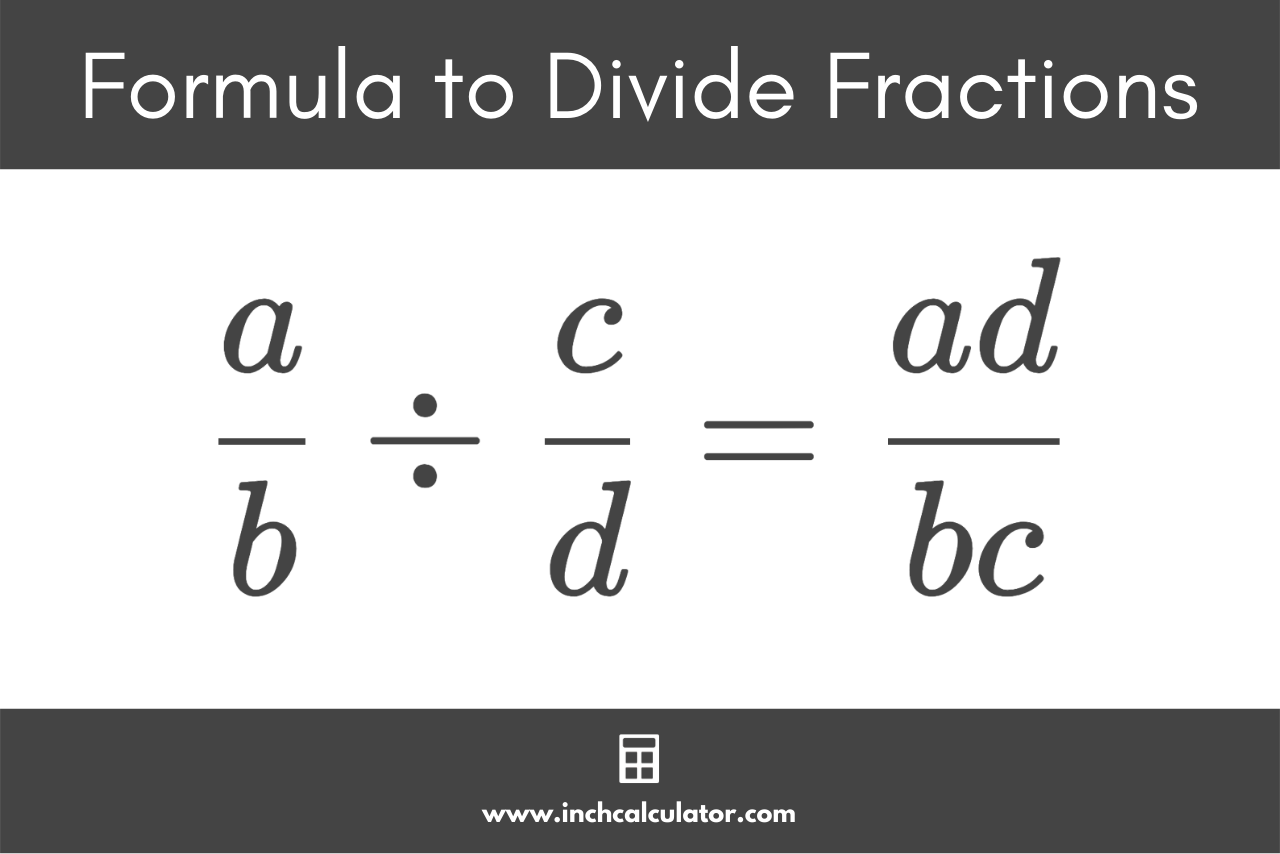The following steps show how to divide fractions using this formula.

### Step One: Multiply Each Numerator by the Opposite Denominator

To divide one fraction by another, start by multiplying the first numerator by the second denominator. Then multiply the second numerator by the first denominator.

For example, let’s divide 2 / 3 by 3 / 4.

2 / 3 ÷ 3 / 4= (2 × 4) / (3 × 3)
2 / 3 ÷ 3 / 4= 8 / 9

### Step Two: Simplify the Fraction

Just like when multiplying fractions, the final step of dividing them is to simplify the fraction. See the instructions to simplify a fraction above.

## How to Calculate Mixed Numbers

Mixed numbers might seem intimidating, but the process of calculating them is nearly the same as a normal fraction with one extra step. As a refresher, a mixed number is a number consisting of a whole number and a proper fraction.

The first thing to do when calculating a mixed number is to remove the whole number and increase the numerator of the fraction to make it an improper fraction.

Start by multiplying the whole number by the denominator.

Then, add the result to the numerator in the remaining fraction. You can always use our mixed number to fraction calculator for this step.

Continue following the steps above to calculate fractions after moving the result to the numerator.

For example, let’s convert the mixed number 2 2 / 5 to an improper fraction.

Multiply the whole number by the denominator.

2 × 5 = 10

Add the result to the numerator.

10 + 2 = 12

Rewrite the fraction.

2 2 / 5 = 12 / 5

## How to Calculate Negative Fractions

A negative fraction is one that is denoted with a minus sign in front. The negative sign can be placed in front of the fraction, aligned with the fraction bar, or it can be in front of the numerator or denominator.

a / b = -a / b = a / -b

When the numerator and the denominator both have a negative sign in front, then the fraction is actually positive. Negative fractions should only have one negative sign.

Because the negative sign can be in several places, it is most helpful to put the sign on the numerator. This allows you to use any of the formulas above to add or subtract a negative fraction.

When you place the negative sign in front of the numerator, you can use any of the formulas or steps above to calculate a fraction normally.